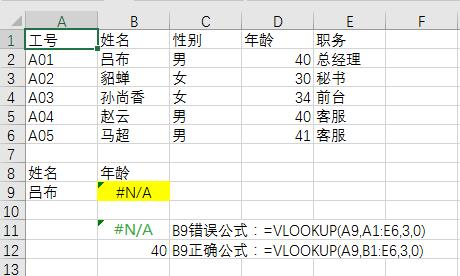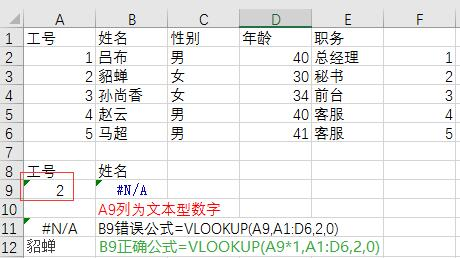# Vlookup函数最常见错误=VLOOKUP(A9,B1:E6,3,0)

【例2】 如下图所示根据姓名查找职务时产生查找错误。=VLOOKUP(A9,B1:E6,4,0)=VLOOKUP(A9,A1:D6,2,0)=VLOOKUP(A9&"",A1:D6,2,0)=VLOOKUP(A9*1,A1:D6,2,0)